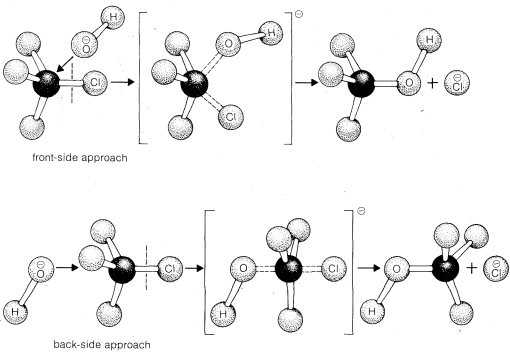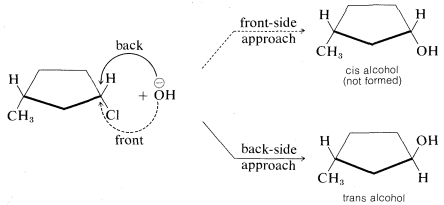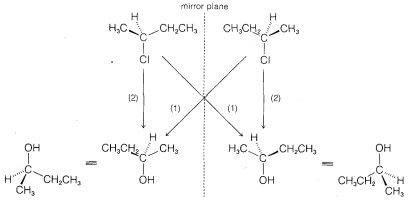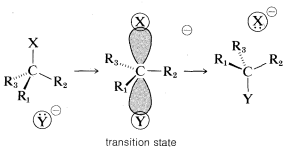# 8.6: Stereochemistry of $$S_N2$$ Reactions

$$\newcommand{\vecs}{\overset { \rightharpoonup} {\mathbf{#1}} }$$ $$\newcommand{\vecd}{\overset{-\!-\!\rightharpoonup}{\vphantom{a}\smash {#1}}}$$$$\newcommand{\id}{\mathrm{id}}$$ $$\newcommand{\Span}{\mathrm{span}}$$ $$\newcommand{\kernel}{\mathrm{null}\,}$$ $$\newcommand{\range}{\mathrm{range}\,}$$ $$\newcommand{\RealPart}{\mathrm{Re}}$$ $$\newcommand{\ImaginaryPart}{\mathrm{Im}}$$ $$\newcommand{\Argument}{\mathrm{Arg}}$$ $$\newcommand{\norm}{\| #1 \|}$$ $$\newcommand{\inner}{\langle #1, #2 \rangle}$$ $$\newcommand{\Span}{\mathrm{span}}$$ $$\newcommand{\id}{\mathrm{id}}$$ $$\newcommand{\Span}{\mathrm{span}}$$ $$\newcommand{\kernel}{\mathrm{null}\,}$$ $$\newcommand{\range}{\mathrm{range}\,}$$ $$\newcommand{\RealPart}{\mathrm{Re}}$$ $$\newcommand{\ImaginaryPart}{\mathrm{Im}}$$ $$\newcommand{\Argument}{\mathrm{Arg}}$$ $$\newcommand{\norm}{\| #1 \|}$$ $$\newcommand{\inner}{\langle #1, #2 \rangle}$$ $$\newcommand{\Span}{\mathrm{span}}$$$$\newcommand{\AA}{\unicode[.8,0]{x212B}}$$

There are two simple ways in which the $$\text{S}_\text{N}2$$ reaction of methyl chloride could occur with hydroxide ion. These differ in the direction of approach of the reagents (Figure 8-1). The hydroxide ion could attack chloromethane at the front side of the carbon where the chlorine is attached or, alternatively, the hydroxide ion could approach the carbon on the side opposite from the chlorine in what is called the back-side approach. In either case, the making of the $$C-O$$ bond is essentially simultaneous with the breaking of the $$C-Cl$$ bond. The difference is that for the back-side mechanism the carbon and the attached hydrogens become planar in the transition state.Figure 8.1: Back-side (inverting) and front-side (noninverting) approach of hydroxide ion on methyl chloride, as visualized with ball-and-stick models

The stereochemical consequences of front- and back-side displacements are different. With cyclic compounds, the two types of displacement lead to different products. For example, an $$\text{S}_\text{N}2$$ reaction between cis-3-methylcyclopentyl chloride and hydroxide ion would give the cis alcohol by front-side approach but the trans alcohol by back-side approach. The actual product is the trans alcohol, from which we know that reaction occurs by back-side displacement:Figure 8-2, demonstrates that front-side displacement of chloride by hydroxide ion will give an enantiomer of 2-butanol of the same configuration as the original chloride, whereas back-side displacement will give the alcohol of the opposite, or inverted, configuration. Experiments using either of the two enantiomers show that hydroxide ion attacks 2-chlorobutane exclusively by back-sideFigure 8.2: Stereochemistry of displacement of 2-chlorobutane with hydroxide by (1) front-side attack (not observed) and (2) back-side attack

displacement to give 2-butanol with the inverted configuration. Similar studies of a wide variety of displacements have established that $$\text{S}_\text{N}2$$ reactions invariably proceed with inversion of configuration via back-side attack. This stereochemical course commonly is known as the Walden inversion.$$^5$$ An orbital picture of the transition state of an $$\text{S}_\text{N}2$$ reaction that leads to inversion of configuration follows:$$^5$$The first documented observation that optically active compounds could react to give products having the opposite configuration was made by P. Walden, in 1895. The implications were not understood, however, until the mechanisms of nucleophilic substitution were elucidated in the 1930's, largely through the work of E. D. Hughes and C. K. Ingold, who established that $$\text{S}_\text{N}2$$ substitutions give products of inverted configuration.

This page titled 8.6: Stereochemistry of $$S_N2$$ Reactions is shared under a CC BY-NC-SA 4.0 license and was authored, remixed, and/or curated by John D. Roberts and Marjorie C. Caserio.